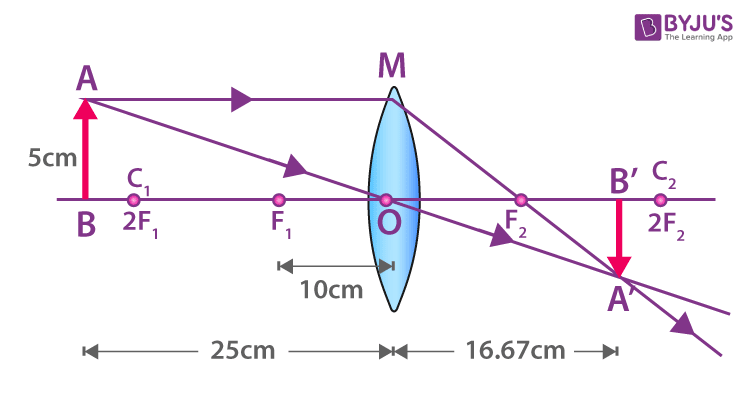# An object 5 cm in length is held 25 cm away from a converging lens of focal length 10 cm. Draw the ray diagram and find the position, size and the nature of the image formed.

Given that

The height of object = 5cm

Position of object, u = – 25cm

The focal length of the lens, f = 10 cm

We need to find

The position of the image, v =?

Size of the image

Nature of the image

Formula

We know that

1/v – 1/u = 1/f

Substituting the known values in the above equation we get,

1/v + 1/25 = 1/10

=> 1/v = 1/10 – 1/25

=> 1/v = (5 – 2)/50

Hence, 1/v = 3/50

So, v= 50/3 = 16.66 cm

Therefore, the distance of the image is 16.66 cm on the opposite side of the lens.

Now, we know that

Magnification = v/u

Hence, m = 16.66/-25 = -0.66

Also, we know that

m= height of image/height of the object

Or, -0.66 = height of image / 5 cm

Hence, height of image = -3.3 cm

The negative sign of the height of the image depicts that an inverted image is formed.

So, the position of image = At 16.66 cm on the opposite side of the lens

Size of image = – 3.3 cm at the opposite side of the lens

Nature of image – Real and inverted4 (345)(1124)(134)

#### Choose An Option That Best Describes Your Problem

Thank you. Your Feedback will Help us Serve you better.